# Boolean differential calculus

(diff) ← Older revision | Latest revision (diff) | Newer revision → (diff)

A branch of mathematics dealing with the concepts of differentials and derivatives of Boolean functions (cf. Boolean function) and the manner of using these in the study of such functions.

Boolean differential calculus originated from the treatment of electrical engineering problems in the areas of error-correcting codes (cf. Error-correcting code) and of design and testing of switching circuits; development into a self-contained mathematical theory was achieved in 1959 [a1], [a2], and continued in the time thereafter [a3], [a4], [a5], [a6]. Boolean differential calculus has also found other engineering applications: e.g., it can be used as a unifying framework for the modeling and investigation of finite automata (cf. Automaton, finite) and of discrete event dynamical systems [a7] (cf. also Discrete event system), i.e., dynamical systems with discrete states and changes of states called events; such systems arise e.g. in digital network communication protocols.

Many concepts in Boolean differential calculus are in analogy to those of classical differential calculus for real-valued functions of one or more real variables; such are, e.g., the concept of a differential, describing the change of the value of a function and variables, and the concept of a derivative, describing how the value of a function depends on changes of its argument(s).

The simplest and (with regard to applications) most important case is based on the two-element Boolean algebra with carrier set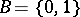, on Boolean or binary variables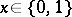, and on vectors of variablesin a Boolean space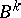. A Boolean functionis a mapping, and a set offunctions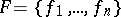can be represented as a mapping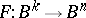.

A Boolean equation of the general form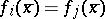can always be written in homogeneous form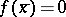, with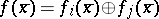, and a set ofsimultaneous equations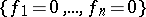can always be combined into one single equation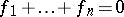. Here and below,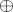denotes addition modulo, or the operation of exclusive or, and the symbols,and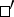stand for disjunction, conjunction and negation, respectively.

## Derivatives.

Supposeand. The (Boolean) derivativeof a Boolean functionwith respect to the variableis the functiongiven by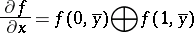or, equivalently,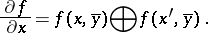It has the valueif and only if a change inchanges the value of.

The maximum and the minimum of the functionwith respect to the variableis defined as:Suppose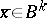and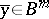. The derivativeof a Boolean function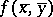with respect to thevariables inis the function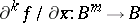,Maxima and minima of a function with respect to more than one of its variables are defined accordingly.

## Differentials.

The variable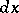defined by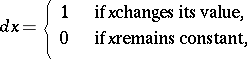is called the differential of the variable, and describes changes in. Likewise, the vectoris called the differential of the vector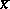, and describes the changes that occur in the components ofwhenchanges to some other value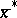; here,denotes component-wise exclusive-or. In,is a point, and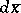is the direction fromto. The (total) differential of a Boolean function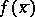is given by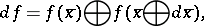the partial differential of a Boolean functionwith respect tois given by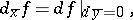and theth partial differential ofwith respect tois given byOther useful operators include the various differential minima and maxima that can be derived from the various differentials of functions by replacing "" with "" or "+" .

Boolean differential equations, as well as Boolean equations, can be solved and investigated with the aid of differential operators. Numerical tools may operate on the solution sets of equations rather than on the equations themselves. A compact representation of solution sets uses-,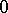-, and "do-not-care" -elements in ternary-valued tables.